# AP Physics C: Electricity and Magnetism Practice Test 6

### Test Information11 questions15 minutes

1. A square conducting loop of wire lies so that the plane of the loop is perpendicular to a constant magnetic field of strength B. Suppose the length of each side of the loop 这是啥 could be increased with time t so that 这是啥 = kt2, where k is a positive constant. What is the magnitude of the emf induced in the loop as a function of time?

2.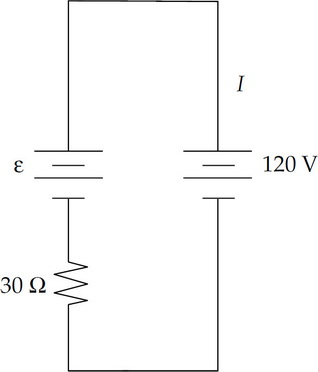A battery with emf ε and internal resistance of 30 Ω is being recharged by connecting it to an outlet with a potential difference of 120 V as shown above. While it is being recharged, 3 A flows through the battery. Determine the emf of the battery.

3.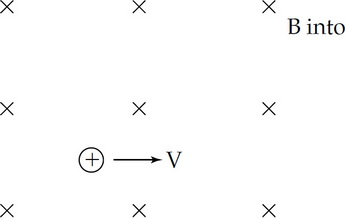A positively charged particle is moving with a constant velocity through a region with both a magnetic field and electric field. The magnetic field and the motion of the particle are shown above. What direction must the electric field be to cause the particle to travel at a constant velocity?

4.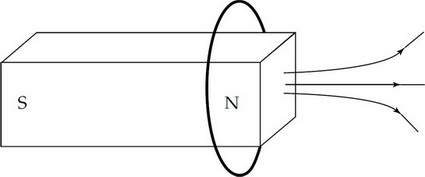A conducting loop of wire is initially around a magnet as shown above. The magnet is moved to the left. What is the direction of the force on the loop and the direction of the magnetic field at the center of the loop due to the induced current?

Direction of Force on Loop &nbps;&nbps;&nbps; Direction of Magnetic Field at Center of Loop Due to Induced Current/b>

5.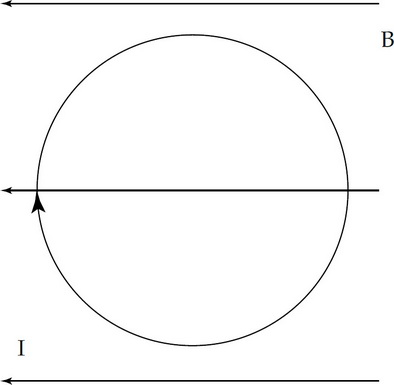A loop of wire carrying a current I is initially in the plane of the page and is located in a uniform magnetic field B which points toward the left side of the page, as shown above. Which of the following shows the correct initial rotation of the loop due to the force exerted by the magnetic field?

6.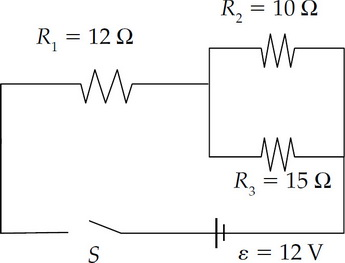What is the equivalent resistance of the three resistors shown in the circuit above?

7.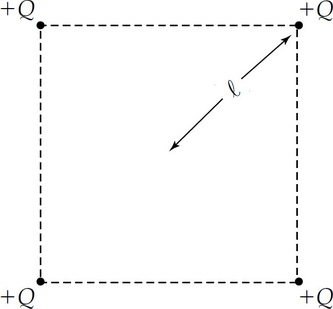Four particles, each with a charge +Q, are held fixed at the corners of a square, as shown above. The distance from each charge to the center of the square is 这是啥.

What is the magnitude of the electric field at the center of the square?

8.Four particles, each with a charge +Q, are held fixed at the corners of a square, as shown above. The distance from each charge to the center of the square is 这是啥.

What is the magnitude of the work required to move a charge of +3Q from the center of the square to very far away?

9.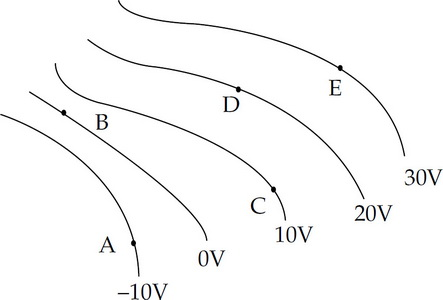The diagram above shows equipotential lines produced by a charge distribution. A, B, C, D, and E are points in the plane.

At which point is the magnitude of the electric field the greatest?

10.The diagram above shows equipotential lines produced by a charge distribution. A, B, C, D, and E are points in the plane.

Which vector below bests describes the direction of the electric field at point D ?

11.The diagram above shows equipotential lines produced by a charge distribution. A, B, C, D, and E are points in the plane.

A particle with a –3 μC charge is released from rest on the –10 V equipotential line. What is the particle's change in electric potential energy when it reaches the 20 V equipotential line?